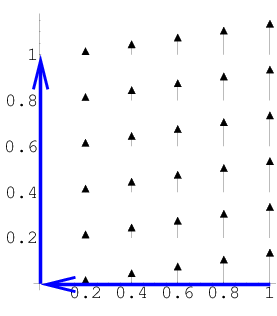# Math Insight

### Image: Example line integral over two line segmentsA line integral of the vector field $\dlvf(x,y) = (0,x)$ is to be taken over a curve composed of two line segments.

Image file: line_integral_vector_example_segments.png